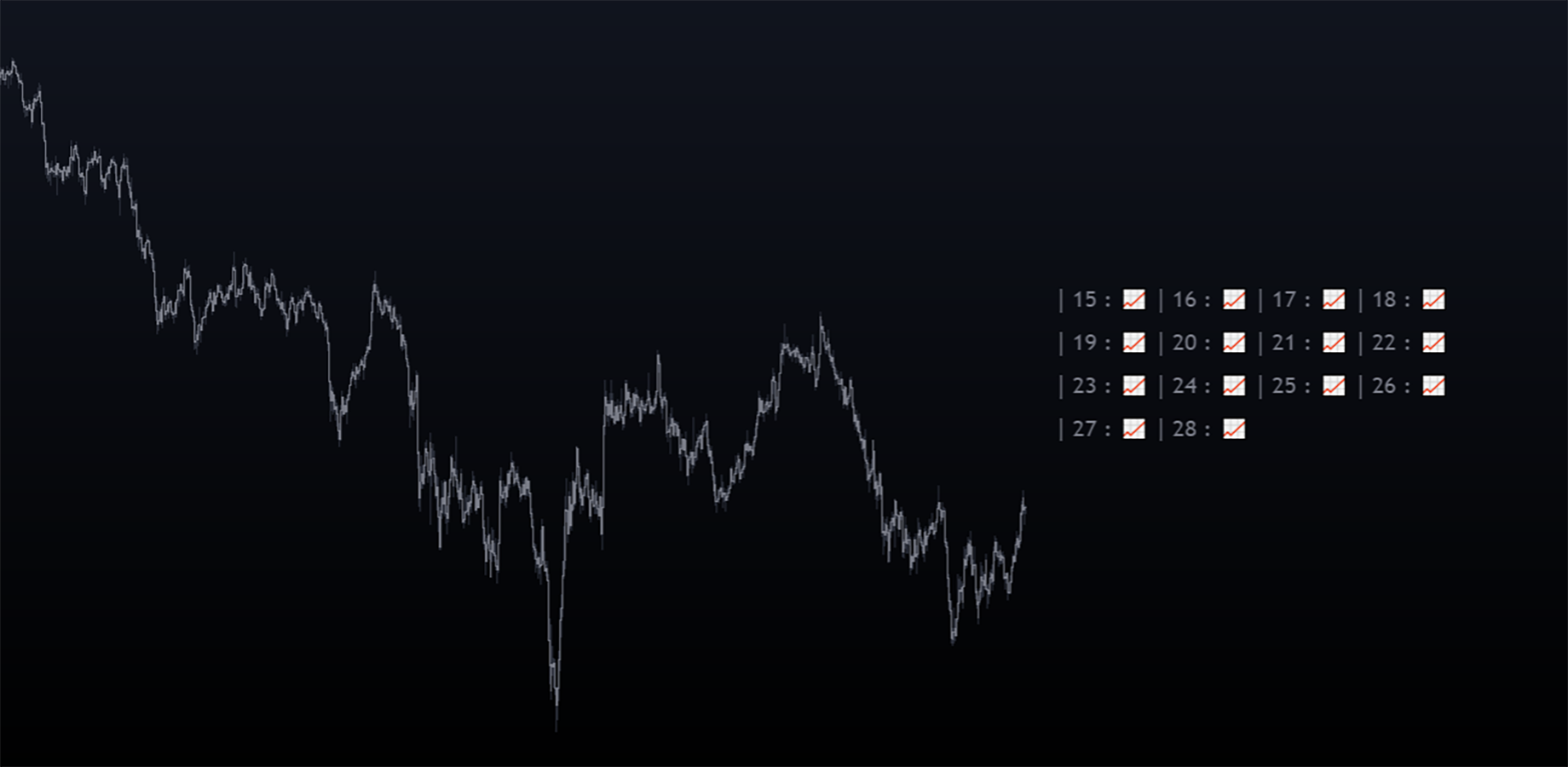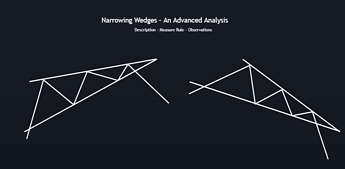# Fibonacci Grid

March 28, 2022

The following indicator returns multiple diagonal lines forming a grid. Each line has 45-degree and is set depending on Fibonacci ratios as well as the maximum and minimum price value over a certain lookback period.

The following indicator returns multiple diagonal lines forming a grid. Each line has 45-degree and is set depending on Fibonacci ratios as well as the maximum and minimum price value over a certain lookback period. These can be used as potential support and resistance .Users also have the option to set equidistant lines instead of having them determined by Fibonacci ratios.

#### Settings

• Length: Lookback period for determining the maximum/minimum price value used for constructing the grid.
• Resolution: Grid resolution, higher values will return more lines (only available when the "Use Fibonacci Ratios" is disabled)
• Use Fibonacci Ratios: Set the lines based on Fibonacci Ratios, 6 ratios are used.

#### Usage

Each individual diagonal line can be used as support/resistance . Two Diagonal lines form channels where the price might evolve until a breakout.The underlying logic of this grid determined by Fibonacci ratios is that price variations farther away from the main diagonals (in orange) would tend to move inside tighter channels.

Diagonals set using Fibonacci Ratios will form tighter channels when away from the center of the Lookback area. While equidistant lines will keep the same distance between each line.

### Other Stories### SMA Directional Matrix - A New Indicator For Technical Analysis

5/13/2022
New Feature
Indicator
Educational

Today we present a new technical indicator that displays a simple & elegant panel showing the direction of simple moving averages with periods in a user-selected range.### Narrowing Wedges - Advanced Analysis

5/13/2022
New Feature
Indicator
Educational

In the last post in this series on chart patterns, we described the characteristics, rules, and causes of broadening wedges patterns (if you haven't seen it, see the related ideas below).